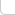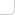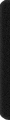# 某岛

… : "…アッカリ～ン . .. . " .. .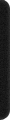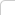September 9, 2015

# ZOJ 2126. Rocket Mania

### Brief description:### Analysis:

```void Init(){

// 1 Left
// 2 Up
// 4 Down
// 8 Right

// '.'
Pattern.PB(0);
// '-'
Pattern.PB(1|8); Pattern.PB(2|4);
// 'L'
Pattern.PB(2|8); Pattern.PB(8|4); Pattern.PB(4|1); Pattern.PB(1|2);
// 'T'
int U = 15;
Pattern.PB(U^1); Pattern.PB(U^2); Pattern.PB(U^4); Pattern.PB(U^8);
// '+';
Pattern.PB(U);
}
```

```
ECH(it, Pattern[A[i][j]]){

decode(H[src].state[ii]);

int p = *it;

int lt = b[j], up = b[j+1];
bool lt2 = _1(p,0) && lt, up2 = _1(p,1) && up;
//需要同时判断格子所在的插头和状态所在的插头同时为真。
bool BurN = ((lt2 && _1(BurNing, j)) || (up2 && _1(BurNing, j+1)));
//并且如果有一处引燃，则生成的新插头也引燃并且如果发生合并则合并过后的插头也。

b[j] = b[j+1] = 0;
BurNing &= ~_1(j);
BurNing &= ~_1(j+1);

int t;

if (lt2 && up2){ // 合并插头，如果有一个引燃则将所有位置等于这两个插头的状态全部置于引燃。
t = up;
REP(k, m+1) if (b[k] == lt || b[k] == up){
if (BurN) BurNing |= _1(k);
b[k] = t;
}
}
else if (lt2 | up2){
if (lt2) t = lt; else t = up;
}
else{ // 注意最多会产生 m 个插头，因而新产生的插头要填 m+1。
t = m+1;
}

if (_1(p,2)){ // 是否产生下插头
b[j] = t;
if (BurN) BurNing |= _1(j);
}

if (_1(p,3) && j != m-1){ // 是否产生右插头
b[j+1] = t;
if (BurN) BurNing |= _1(j+1);
}

if (!BurNing) continue; // Cut-1

RST(bb); REP(k, m+1) if (b[k]) ++bb[b[k]];
REP(k, m+1) if (bb[b[k]] == 1 && !_1(BurNing, k)) b[k] = 0; // Cut-2

LL s = encode(); _des.push_back(s);
if (count_bits(BurNing) > best){
best = count_bits(BurNing);
critical = s;
}
}
```

• 剪枝一：如果轮廓线上所有的插头全部都未被点燃，那么最后所有的火箭都不可能发射，所以这个状态可以舍去．这个剪枝看上去非常显然，对于大部分数据却可以剪掉近乎一半的状态．
• 剪枝二：如果轮廓线上有一个插头p，它没有被火柴点燃且没有其它的插头与它连通，那么这个插头可以认为是“无效”插头．因为即使这个插头所在的路径以后会被点燃而可以发射某个火箭，那么一定存在另一条路径可以不经过这个插头而发射火箭，如图．这种情况下将插头设置为不存在．这是最重要的一个剪枝，大部分数据的状态总数可以缩小七八倍，甚至十几倍．
• 剪枝三：这是一个最优性问题，我们考虑最优性剪枝：对于一个格子(i, j)的两个状态 (S0, Fired0)， (S1, Fired1)，如果第一个状态的每一个存在的插头在第二个状态中不仅存在而且都被点燃，那么无论以后如何决策，第二个状态点燃的火箭个数不会少于第一个状态，这样我们就可以果断地舍去第一个状态．对于每一个(i, j)，选择Ones[Fired]最多的一个状态Best，如果一个状态一定不比Best好，就可以舍去．

• 剪枝四：从边界情况入手，边界状态非常特殊，也非常容易导致产生无效状态．分析一下，转移完最后一列的某个格子(i, 6)后，如果I类插头中某个插头p没有被点燃，并且II类插头中没有插头与它连通，那么这个插头就成了“无效”插头．

https://gist.github.com/lychees/085c994a7bedb29154bd